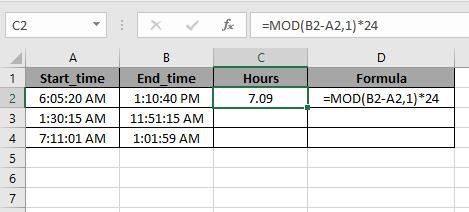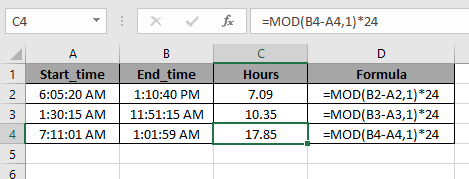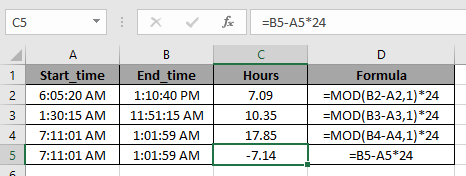# How to take Time difference in hours as decimal value in Excel

In this article, we will learn about how to calculate the Time difference in hours as decimal values in Excel.

In simple words, We need to get the time difference in hours using Excel MOD function and then we need to represent it in the decimal format.

MOD function returns the remainder when number gets divided by divisor.
Syntax for MOD function:

=MOD(number, divisor)

Number : The number to be divided
Divisor : The number to divide with.
Let’s understand this function using it an example.

Here we have some time fields named Start_time & End_time.We need to calculate the time difference using MOD function. And then we will multiple by 24 to get the fraction value to hours in decimal value.
Use the formula to get positive Hours in decimal values:

=MOD( B2-A2 , 1 ) * 24

Explanation:
B2-C2: takes the difference between the two times.
MOD function solves the problem of negative answers.
*24 : The number gets multiplied with 24 to get the hours in decimal valuesCopy the formula in other cells using shortcut key Ctrl + D.As you can see the MOD function gets the hours in decimal values and returns no result in negative hours.

Note:
You can also do the above process via just taking the difference and multiply the result with 24 to get hours in decimal value.But here MOD function saved us the trouble of negative Hours.

Hope you understood how to take Time difference in hours as decimal values in Excel. Explore more articles on Excel Date & Time function here. Please feel free to state your query or feedback for the above article.

Related Articles:

How to Convert Date and Time from GMT to CST in Excel

How to Calculate Minutes Between Dates & Time In Microsoft Excel

How to Calculate hours between time in Excel

How to convert decimal Seconds into time format in Excel

How to Convert Time to Decimal in Excel

Popular Articles

50 Excel Shortcut to Increase Your Productivity : Get faster at your task. These 50 shortcuts will make you work even faster on Excel.

How to use the VLOOKUP Function in Excel : This is one of the most used and popular functions of excel that is used to lookup value from different ranges and sheets.

How to use the COUNTIF function in Excel : Count values with conditions using this amazing function. You don't need to filter your data to count specific values. Countif function is essential to prepare your dashboard.

How to use the SUMIF Function in Excel : This is another dashboard essential function. This helps you sum up values on specific conditions.

Terms and Conditions of use

The applications/code on this site are distributed as is and without warranties or liability. In no event shall the owner of the copyrights, or the authors of the applications/code be liable for any loss of profit, any problems or any damage resulting from the use or evaluation of the applications/code.